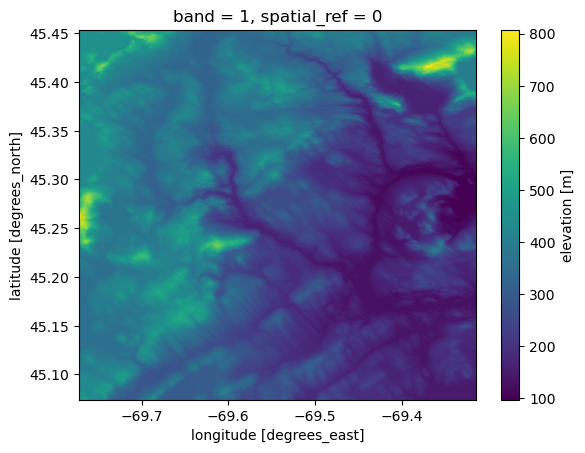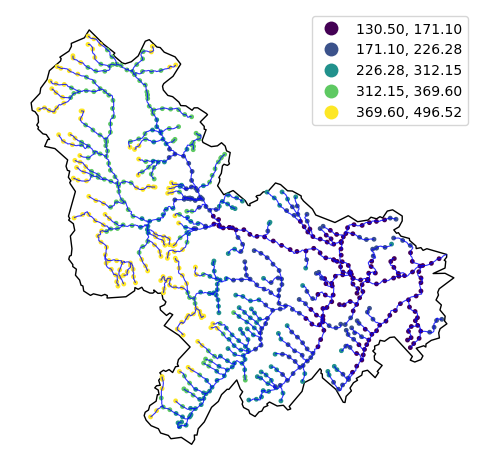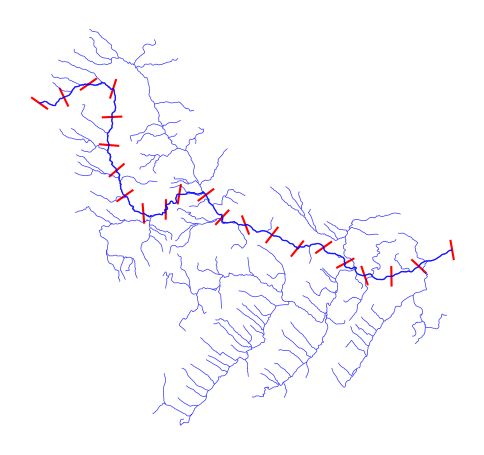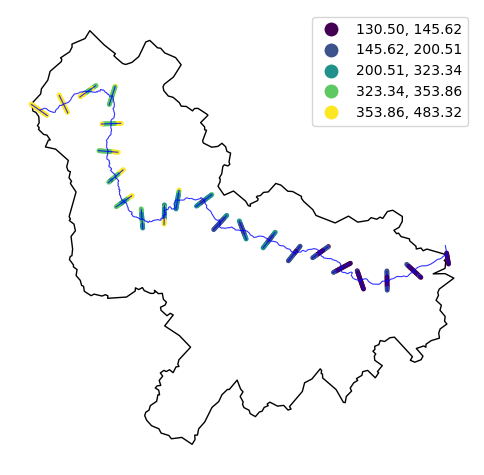This page was generated from cross_section.ipynb. Interactive online version:# River Elevation and Cross-Section#

:

from pathlib import Path

import geopandas as gpd
import numpy as np
import pandas as pd
from shapely.geometry import Point

import py3dep
import pygeoogc.utils as ogc_utils
import pynhd
from pynhd import NLDI, WaterData

:

import warnings

warnings.filterwarnings("ignore", message=".*Index.*")


We can retrieve elevation profile for tributaries of a given USGS station ID using PyNHD and Py3DEP. For this purpose, we get the elevation data for points along the tributaries’ flowlines every one kilometer. Note that since the distance is in meters we reproject the geospatial data into ESRI:102003.

:

CRS = "ESRI:102003"

station_id = "01031500"
distance = 1000  # in meters


First, let’s get the basin geometry and tributaries for the station.

:

nldi = NLDI()

basin = nldi.get_basins(station_id)
flw = nldi.navigate_byid(
fsource="nwissite",
fid=f"USGS-{station_id}",
source="flowlines",
distance=1000,
)
flw = flw.set_index("nhdplus_comid").to_crs(CRS)


Now, we can compute the number of points along each river segment based on the target 1-km distance. Note that since we want to sample the data from a DEM in EPSG:4326 wen need to reproject the sample points from EPSG:102003 to EPSG:4326. We use pygeoogc.utils.match_crs to do so.

:

flw["n_points"] = np.ceil(flw.length / distance).astype("int")
flw_points = [
(
n,
ogc_utils.match_crs(
[
(p, p)
for p in (
l.interpolate(x, normalized=True).xy
for x in np.linspace(0, 1, pts, endpoint=False)
)
],
CRS,
"EPSG:4326",
),
)
for n, l, pts in flw.itertuples(name=None)
]


We use Py3DEP to get elevation data for these points. We can either use py3dep.elevation_bycoords to get elevations directly from the Elevation Point Query Service (EPQS) at 10-m resolution or use py3dep.get_map to get the elevation data for the entire basin and then sample it for the target locations.

Using py3dep.elevation_bycoords is simpler but the EPQS service is a bit unstable and slow. Here’s the code to get the elevations using EPQS:

flw_elevation = [(n, py3dep.elevation_bycoords(pts, CRS)) for n, pts in flw_points]


Let’s use py3dep.get_map instead since we can control the resolution and reuse it later on.

:

dem = py3dep.get_map("DEM", basin.geometry.bounds, resolution=10)


Additionally, if pyflowdir is installed, we can use py3dep.fill_depressions function for filling its depressions.

:

dem = py3dep.fill_depressions(dem)
dem.plot()

:

<matplotlib.collections.QuadMesh at 0x1affc2920>:

flw_elevation = [
(n, [dem.interp(x=[x], y=[y]).values for x, y in pts]) for n, pts in flw_points
]


Now that we have both the coordinates and their elevation data for the points, we can create a new dataframe and plot the results.

:

points = pd.DataFrame(flw_points).set_index(0).explode()
points = pd.DataFrame([(n, Point(p)) for n, p in points.items()])
points = points.rename(columns={0: "comid", 1: "geometry"}).set_index("comid")
points = gpd.GeoDataFrame(points, crs="EPSG:4326").to_crs(CRS)
elevations = pd.DataFrame(flw_elevation).rename(columns={0: "comid", 1: "elevation"})
elevations = elevations.set_index("comid")["elevation"].explode().astype("float")
points["elevation"] = elevations

:

ax = basin.to_crs(CRS).plot(figsize=(6, 6), facecolor="none", edgecolor="black")
points.plot(ax=ax, column="elevation", markersize=6, legend=True, scheme="quantiles")
flw.plot(ax=ax, color="b", linewidth=0.8, alpha=0.8)
ax.set_axis_off()Let’s extract corss-section profiles along the main river of the basin. We get cross-section profiles at every 3 km along the main river and within a buffer distance of 2 km.

:

distance = 2000  # in meters
width = 2000  # in meters
half_width = width * 0.5


First, we need to get the main river’s flowlines.

:

main = nldi.navigate_byid(
fsource="nwissite",
fid=f"USGS-{station_id}",
source="flowlines",
distance=1000,
)
main = main.set_index("nhdplus_comid").to_crs(CRS)

:

nhd = WaterData("nhdflowline_network")
main_nhd = nhd.byid("comid", main.index.to_list())
main_nhd = pynhd.prepare_nhdplus(main_nhd, 0, 0, 0, purge_non_dendritic=True).to_crs(CRS)
cs = pynhd.network_xsection(main_nhd, distance, width)


Let’s plot the obtained cross-section lines.

:

ax = cs.plot(color="r", figsize=(6, 6))
main.plot(ax=ax, color="b", linewidth=1, alpha=0.8)
flw.plot(ax=ax, color="b", linewidth=0.5, alpha=0.8)
ax.set_axis_off()
ax.figure.savefig(Path("_static", "cross_section.png"), dpi=300, bbox_inches="tight", facecolor="w")Using the obtained cross-section lines, we can compute the cross-section profiles every 500 meters along the cross-section lines following the same procedure as before.

:

distance = 100  # in meters
cs["n_points"] = np.ceil(cs.length / distance).astype("int")
cs_points = [
(
n,
ogc_utils.match_crs(
[
(p, p)
for p in (
l.interpolate(x, normalized=True).xy
for x in np.linspace(0, 1, pts, endpoint=False)
)
],
CRS,
"EPSG:4326",
),
)
for n, l, pts in cs.itertuples(name=None)
]
cs_elevation = [
(n, [dem.interp(x=[p], y=[p]).values for p in pts]) for n, pts in cs_points
]

:

points = pd.DataFrame(cs_points).set_index(0).explode()
points = pd.DataFrame([(n, Point(p)) for n, p in points.items()])
points = points.rename(columns={0: "comid", 1: "geometry"}).set_index("comid")
points = gpd.GeoDataFrame(points, crs="EPSG:4326").to_crs(CRS)
elevations = pd.DataFrame(cs_elevation).rename(columns={0: "comid", 1: "elevation"})
elevations = elevations.set_index("comid")["elevation"].explode().astype("float")
points["elevation"] = elevations

:

ax = basin.to_crs(CRS).plot(figsize=(6, 6), facecolor="none", edgecolor="black")
main.plot(ax=ax, color="b", linewidth=0.8, alpha=0.8)
points.plot(ax=ax, column="elevation", markersize=6, legend=True, scheme="quantiles")
cs.plot(ax=ax, color="b", linewidth=0.8, alpha=0.8)
ax.set_axis_off()